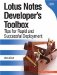# Working with Operators

Operators are used to compare or equate values and for mathematic calculations. The following table lists the primary operators used in formulas.

Operator

Operator Usage or Description

-

Used in subtraction or to represent a negative number

!

Represents a logical NOT

!=

Represents not equal

&

Represents a logical AND

*

Used in multiplication

/

Used in division

:

Used to separate a list of values

:=

Used to assign a value to a variable

|

Represents a logical OR

+

Used in addition or to represent a positive number

<

Represents less than

<=

Represents less than or equal to

<>

Represents not equal

=

Represents equal

=!

Represents not equal

>

Represents greater than

><

Represents not equal

>=

Represents greater than or equal toLotus Notes Developers Toolbox: Tips for Rapid and Successful Deployment
ISBN: 0132214482
EAN: 2147483647
Year: N/A
Pages: 293
Authors: Mark Elliott